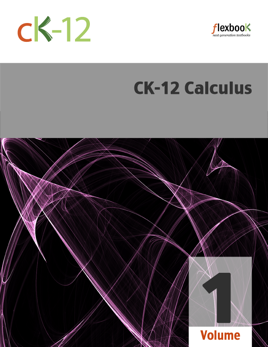## Publisher Description

CK-12 Foundation’s Calculus, Volume 1 FlexBook covers the following four chapters:
Functions, Limits, and Continuity – A review of the basics of functions is given. Students use linear approximations to study the limit process, before a more formal treatment of limits is given.
Differentiation – Students explore instantaneous rate of change, and the relationship between continuity and differentiability. The Chain Rule and implicit differentiation are reviewed.
Applications of Derivatives – Students gain practice with using the derivatives in related rates problems. Additional topics include The First Derivative Test, The Second Derivative Test, limits at infinity, optimization, and approximation errors.
Integration – This chapter includes indefinite integrals calculus, initial value problems, definite integrals, the Fundamental Theorem of Calculus, integration by substitution, and numerical integration.